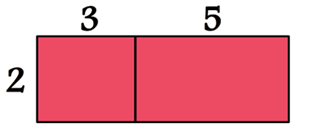Mathematics
Easy

Question

# The following expressions represent the total area of the rectangle is?## 5(2 + 3)    3(2 + 5)    2(3 + 5)    2(3) + 3(5)Hint:

## The correct answer is: 2(3 + 5)

### In the it is given that,The length of the rectangle = (3+5) unitThe breadth of the rectangle = 2 unitHere, we have to find the area of the given rectangle.As the area of the rectangle will be length × breadth.So, the expression will be 2(3+5) unit.Therefore, the correct option is c, i.e., 2(3+5).

In distributive property, when a factor is to be multiplied with the sum of two numbers, we do not directly add the two numbers and then multiply the sum with the factor. Rather, we multiply both the numbers with the factor differently and then add the multiplication value.

### Related Questions to study#### With Turito Foundation.#### Get an Expert Advice From Turito.